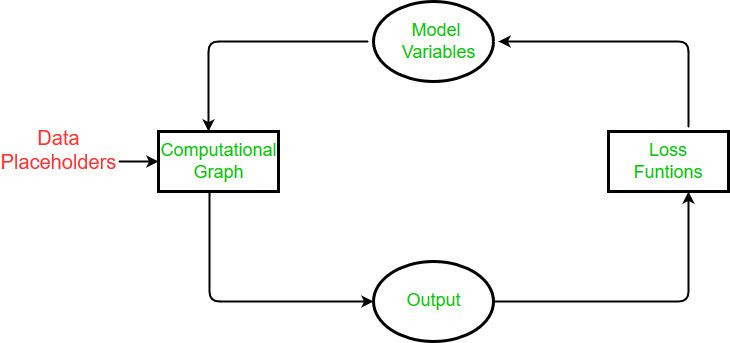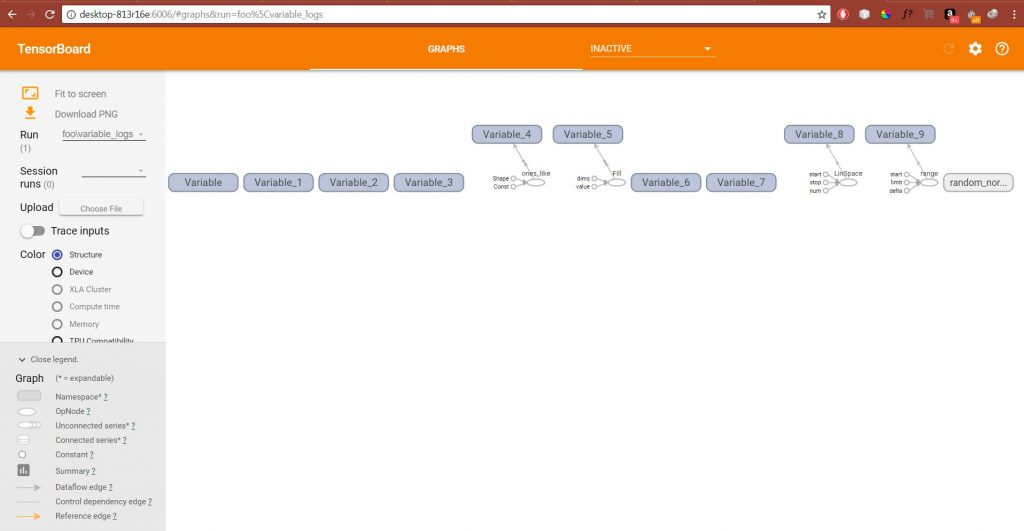# Introduction to Tensor with Tensorflow

• Last Updated : 06 Dec, 2020

TensorFlow is an open-source software library for dataflow programming across a range of tasks. It is a symbolic math library, and also used for machine learning applications such as neural networks. Google open-sourced TensorFlow in November 2015. Since then, TensorFlow has become the most starred machine learning repository on Github. (https://github.com/tensorflow/tensorflow)

Why TensorFlow? TensorFlow’s popularity is due to many things, but primarily because of the computational graph concept, automatic differentiation, and the adaptability of the Tensorflow python API structure. This makes solving real problems with TensorFlow accessible to most programmers.

Google’s Tensorflow engine has a unique way of solving problems. This unique way allows for solving machine learning problems very efficiently. We will cover the basic steps to understand how Tensorflow operates.

What is Tensor in Tensorflow

TensorFlow, as the name indicates, is a framework to define and run computations involving tensors. A tensor is a generalization of vectors and matrices to potentially higher dimensions. Internally, TensorFlow represents tensors as n-dimensional arrays of base datatypes. Each element in the Tensor has the same data type, and the data type is always known. The shape (that is, the number of dimensions it has and the size of each dimension) might be only partially known. Most operations produce tensors of fully-known shapes if the shapes of their inputs are also fully known, but in some cases it’s only possible to find the shape of a tensor at graph execution time.

### General TensorFlow Algorithm Outlines

Here we will introduce the general flow of Tensorflow Algorithms.

1. Import or generate data

All of our machine learning algorithms will depend on data. In practice, we will either generate data or use an outside source of data. Sometimes it is better to rely on generated data because we will want to know the expected outcome. And also tensorflow comes preloaded with famous datasets like MNIST, CIFAR-10, etc.

2. Transform and normalize data

The data is usually not in the correct dimension or type that our Tensorflow algorithms expect. We will have to transform our data before we can use it. Most algorithms also expect normalized data. Tensorflow has built in functions that can normalize the data for you.

`data = tf.nn.batch_norm_with_global_normalization(...)`
3. Set algorithm parameters

Our algorithms usually have a set of parameters that we hold constant throughout the procedure. For example, this can be the number of iterations, the learning rate, or other fixed parameters of our choosing. It is considered good form to initialize these together so the reader or user can easily find them.

`learning_rate = 0.001  iterations = 1000`
4. Initialize variables and placeholders

Tensorflow depends on us telling it what it can and cannot modify. Tensorflow will modify the variables during optimization to minimize a loss function. To accomplish this, we feed in data through placeholders. We need to initialize both of these, variables and placeholders with size and type, so that Tensorflow knows what to expect.

`a_var = tf.constant(42)  x_input = tf.placeholder(tf.float32, [None, input_size])  y_input = tf.placeholder(tf.fload32, [None, num_classes])`
5. Define the model structure

After we have the data, and initialized our variables and placeholders, we have to define the model. This is done by building a computational graph. We tell Tensorflow what operations must be done on the variables and placeholders to arrive at our model predictions.

`y_pred = tf.add(tf.mul(x_input, weight_matrix), b_matrix)`
6. Declare the loss functions

After defining the model, we must be able to evaluate the output. This is where we declare the loss function. The loss function is very important as it tells us how far off our predictions are from the actual values.

`loss = tf.reduce_mean(tf.square(y_actual – y_pred))`
7. Initialize and train the model

Now that we have everything in place, we create an instance or our graph and feed in the data through the placeholders and let Tensorflow change the variables to better predict our training data. Here is one way to initialize the computational graph.

```with tf.Session(graph=graph) as session:
...
session.run(...)
...```

Note that we can also initiate our graph with

`session = tf.Session(graph=graph)  session.run(…)`
8. Evaluate the model(Optional)

Once we have built and trained the model, we should evaluate the model by looking at how well it does on new data through some specified criteria.

9. Predict new outcomes(Optional)

It is also important to know how to make predictions on new, unseen, data. We can do this with all of our models, once we have them trained.

### Summary

In Tensorflow, we have to setup the data, variables, placeholders, and model before we tell the program to train and change the variables to improve the predictions. Tensorflow accomplishes this through the computational graph. We tell it to minimize a loss function and Tensorflow does this by modifying the variables in the model. Tensorflow knows how to modify the variables because it keeps track of the computations in the model and automatically computes the gradients for every variable. Because of this, we can see how easy it can be to make changes and try different data sources.

Overall, algorithms are designed to be cyclic in TensorFlow. We set up this cycle as a computational graph and (1) feed in data through the placeholders, (2) calculate the output of the computational graph, (3) compare the output to the desired output with a loss function, (4) modify the model variables according to the automatic back propagation, and finally (5) repeat the process until a stopping criteria is met.Now starts the practical session with tensorflow and implementing tensors using it.

First, we need to import the required libraries.

```import tensorflow as tf
from tensorflow.python.framework import ops
ops.reset_default_graph()```

Then to start the graph session

`sess = tf.Session()`

Now comes the main part i.e. to create tensors.

TensorFlow has built in function to create tensors for use in variables. For example, we can create a zero filled tensor of predefined shape using the tf.zeros() function as follows.

`my_tensor = tf.zeros([1,20])`

We can evaluate tensors with calling a run() method on our session.

`sess.run(my_tensor)`

TensorFlow algorithms need to know which objects are variables and which are constants. So we create a variable using the TensorFlow function tf.Variable(). Note that you can not run sess.run(my_var), this would result in an error. Because TensorFlow operates with computational graphs, we have to create a variable initialization operation in order to evaluate variables. For this script, we can initialize one variable at a time by calling the variable method my_var.initializer.

```my_var = tf.Variable(tf.zeros([1,20]))
sess.run(my_var.initializer)
sess.run(my_var)
```

Output:

```array([[ 0.,  0.,  0.,  0.,  0.,  0.,  0.,
0.,  0.,  0.,  0.,  0.,  0.,  0.,
0.,  0.,  0.,  0.,  0.,  0.]], dtype=float32)```

Now let’s create our variable to handle dimensions of having specific shape then initialize the variables with all ‘1’ or ‘0’

```row_dim = 2
col_dim = 3
zero_var = tf.Variable(tf.zeros([row_dim, col_dim]))
ones_var = tf.Variable(tf.ones([row_dim, col_dim]))
```

Now evaluate the values of them we can run initializer methods on our variables again.

```sess.run(zero_var.initializer)
sess.run(ones_var.initializer)
print(sess.run(zero_var))
print(sess.run(ones_var))```

Output:

```[[ 0.  0.  0.]
[ 0.  0.  0.]]
[[ 1.  1.  1.]
[ 1.  1.  1.]]```

And this list will go on. The rest will be for you study, follow this jupyter notebook by me to get more information about the tensors from here.

Visualizing the Variable Creation in TensorBoard

To visualize the creation of variables in Tensorboard, we will reset the computational graph and create a global initializing operation.

 `# Reset graph``ops.reset_default_graph()`` ` `# Start a graph session``sess ``=` `tf.Session()`` ` `# Create variable``my_var ``=` `tf.Variable(tf.zeros([``1``,``20``]))`` ` `# Add summaries to tensorboard``merged ``=` `tf.summary.merge_all()`` ` `# Initialize graph writer:``writer ``=` `tf.summary.FileWriter(``"/tmp/variable_logs"``, graph``=``sess.graph)`` ` `# Initialize operation``initialize_op ``=` `tf.global_variables_initializer()`` ` `# Run initialization of variable``sess.run(initialize_op)`

Now run the following command in cmd.

`tensorboard --logdir=/tmp`

And it will tell us the URL we can navigate our browser to see Tensorboard, to achieve your loss graphs.

Code to create all types of tensor and evaluate them.

## Recommended: Please try your approach on {IDE} first, before moving on to the solution.

 `import` `tensorflow as tf``from` `tensorflow.python.framework ``import` `ops``ops.reset_default_graph()`` ` `# Introduce tensors in tf`` ` `# Get graph handle``sess ``=` `tf.Session()`` ` `my_tensor ``=` `tf.zeros([``1``,``20``])`` ` `# Declare a variable``my_var ``=` `tf.Variable(tf.zeros([``1``,``20``]))`` ` `# Different kinds of variables``row_dim ``=` `2``col_dim ``=` `3` ` ` `# Zero initialized variable``zero_var ``=` `tf.Variable(tf.zeros([row_dim, col_dim]))`` ` `# One initialized variable``ones_var ``=` `tf.Variable(tf.ones([row_dim, col_dim]))`` ` `# shaped like other variable``sess.run(zero_var.initializer)``sess.run(ones_var.initializer)``zero_similar ``=` `tf.Variable(tf.zeros_like(zero_var))``ones_similar ``=` `tf.Variable(tf.ones_like(ones_var))`` ` `sess.run(ones_similar.initializer)``sess.run(zero_similar.initializer)`` ` `# Fill shape with a constant``fill_var ``=` `tf.Variable(tf.fill([row_dim, col_dim], ``-``1``))`` ` `# Create a variable from a constant``const_var ``=` `tf.Variable(tf.constant([``8``, ``6``, ``7``, ``5``, ``3``, ``0``, ``9``]))``# This can also be used to fill an array:``const_fill_var ``=` `tf.Variable(tf.constant(``-``1``, shape``=``[row_dim, col_dim]))`` ` `# Sequence generation``linear_var ``=` `tf.Variable(tf.linspace(start``=``0.0``, stop``=``1.0``, num``=``3``)) ``# Generates [0.0, 0.5, 1.0] includes the end`` ` `sequence_var ``=` `tf.Variable(tf.``range``(start``=``6``, limit``=``15``, delta``=``3``)) ``# Generates [6, 9, 12] doesn't include the end`` ` `# Random Numbers`` ` `# Random Normal``rnorm_var ``=` `tf.random_normal([row_dim, col_dim], mean``=``0.0``, stddev``=``1.0``)`` ` `# Add summaries to tensorboard``merged ``=` `tf.summary.merge_all()`` ` `# Initialize graph writer:`` ` `writer ``=` `tf.summary.FileWriter(``"/tmp/variable_logs"``, graph``=``sess.graph)`` ` `# Initialize operation``initialize_op ``=` `tf.global_variables_initializer()`` ` `# Run initialization of variable``sess.run(initialize_op)`

Output: